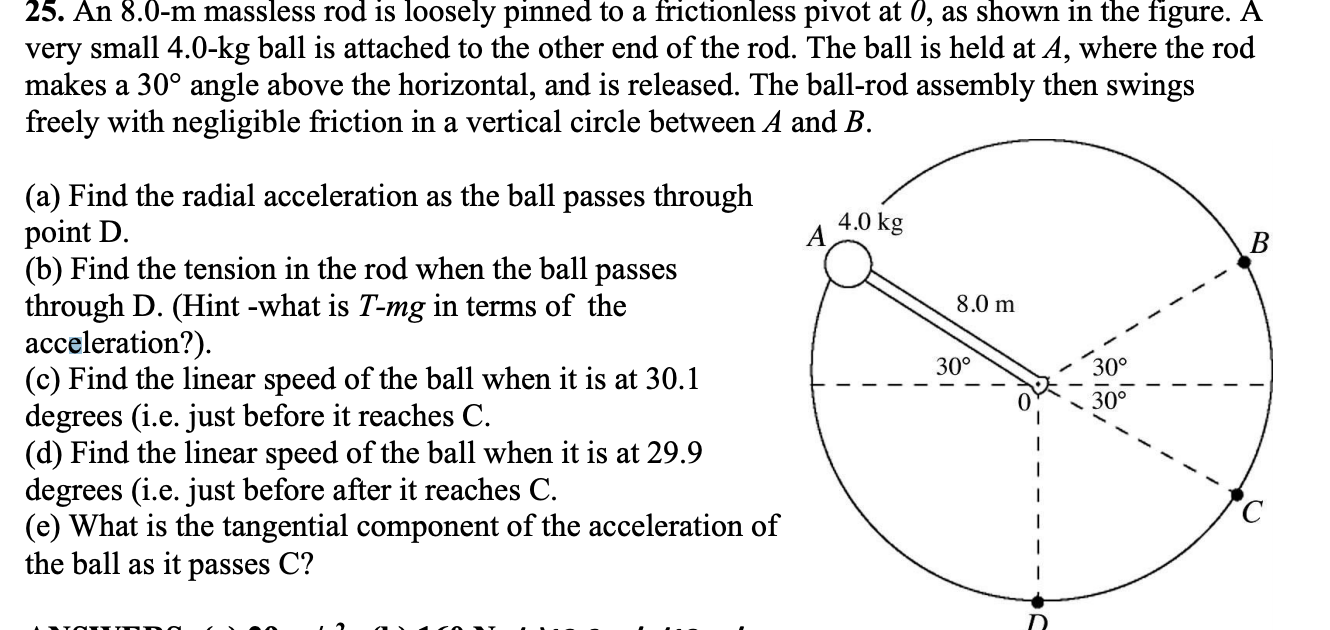# 25. An 8.0-m massless rod is loosely pinned to a frictionless pivot at 0, as shown in the figure. Avery small 4.0-kg ball is attached to the other end of the rod. The ball is held at A, where the rodmakes a 30° angle above the horizontal, and is released. The ball-rod assembly then swingsfreely with negligible friction in a vertical circle between A and B.(a) Find the radial acceleration as the ball passes throughpoint D.(b) Find the tension in the rod when the ball passesthrough D. (Hint -what is T-mg in terms of theacceleration?).(c) Find the linear speed of the ball when it is at 30.1degrees (i.e. just before it reaches C.(d) Find the linear speed of the ball when it is at 29.9degrees (i.e. just before after it reaches C.(e) What is the tangential component of the acceleration ofthe ball as it passes C?4.0 kg8.0 m30°

Question
39 views

I need the solution for the question attached. Thankshelp_outlineImage Transcriptionclose25. An 8.0-m massless rod is loosely pinned to a frictionless pivot at 0, as shown in the figure. A very small 4.0-kg ball is attached to the other end of the rod. The ball is held at A, where the rod makes a 30° angle above the horizontal, and is released. The ball-rod assembly then swings freely with negligible friction in a vertical circle between A and B. (a) Find the radial acceleration as the ball passes through point D. (b) Find the tension in the rod when the ball passes through D. (Hint -what is T-mg in terms of the acceleration?). (c) Find the linear speed of the ball when it is at 30.1 degrees (i.e. just before it reaches C. (d) Find the linear speed of the ball when it is at 29.9 degrees (i.e. just before after it reaches C. (e) What is the tangential component of the acceleration of the ball as it passes C? 4.0 kg 8.0 m 30° fullscreen
check_circle

Step 1

(c)

According to the law of conservation of energy, the linear speed of the ball is,

Substitute the values,

Step 2

### Want to see the full answer?

See Solution

#### Want to see this answer and more?

Solutions are written by subject experts who are available 24/7. Questions are typically answered within 1 hour.*

See Solution
*Response times may vary by subject and question.
Tagged in

### Newtons Laws of Motion